### Home > CAAC > Chapter 10 > Lesson 10.3.1 > Problem10-105

10-105.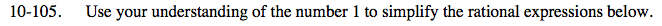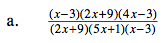Simplify by finding factors that make 'one'.

$\frac{(x-3)(2x+9)(4x-3)}{(2x+9)(5x+1)(x-3)}$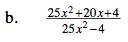Factor the expression, then use the same method as in part (a) to solve.

$\frac{(5x+2)(5x+2)}{(5x-2)(5x+2)}$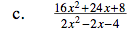Factor the expression, then use the same method as part (a).

$\frac{4(2x+1)}{(x-2)}$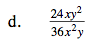Find common factors of the numerator and the denominator that make 'one'.

$\frac{24xy^2}{36x^2y}=\frac{12}{12}\cdot\frac{2}{3}\cdot\frac{x}{x}\cdot\frac{1}{x}\cdot\frac{y}{y}\cdot\frac{y}{1}=\frac{2y}{3x}$